## Newton's Laws Applications Review

#### Navigate to:

Review Session Home - Topic Listing

Forces in 2 Dimensions - Home || Printable Version || Questions with Links

Answers to Questions:  All || #1-12 || #13-21 || #22-30

 [ #1 | #2 | #3 | #4 | #5 | #6 | #7 | #8 | #9 | #10 | #11 | #12 | #13 | #14 | #15 | #16 | #17 | #18 | #19 | #20 | #21 | #22 | #23 | #24 | #25 | #26 | #27 | #28 | #29 | #30 ]

### Part A: Drawing Free-Body Diagrams

1. Construct free-body diagrams for the following physical situations.

 a. A ball is dropped from rest from the top of a building. Assume negligible air resistance.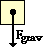In the absence of Fair, the only force acting upon the ball is gravity. It is a projectile. b. After being thrown, a football is moving upwards and rightwards towards the peak of its trajectory. Assume negligible air resistance.In the absence of Fair, the only force acting upon the ball is gravity. It is a projectile. Note that an upwards moving object does not need an upwards force. Only an upwards accelerating object requires an upwards force. c. After reaching a terminal velocity, a falling skydiver then opens up the parachute.When the terminal velocity was reached, Fair = Fgrav. Then the parachute was opened, making Fair even greater than before. This is represented by the larger arrow. d. An air track glider is gliding to the right at constant velocity.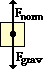There is no rightwards force. A rightwards force would only be required if there is a rightwards acceleration. If the glider is gliding; there is no mention of it being pushed or pulled (Fapp) and if there is a constant velocity, there must be balanced forces. e. A car is skidding to a stop while travling to the right.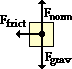There is no rightwards force. A rightwards force would only be required if there is a rightwards acceleration. If the car is skidding (wheels are locked), friction acts in a direction opposite its motion to slow it down. f. A downward moving elevator (held by a cable) slows down.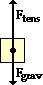The cable supplies the tension force. Since the elevator is moving downwards and slowing down, there must be more upwards force than the downwards gravity force. g. A 25.0-N force is applied at a 30-degree angle to a crate in order to accelerate it rightward across a rough, horizontal surface.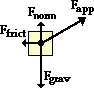The applied force is upwards and rightwards. Its upward component contributes to the upwards Fnorm to balance the force of gravity. (Note the relative size of Fnorm.) h. A picture hangs summetrically by two wires oriented at angles to the vertical.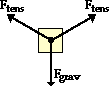The force exerted by a wire is a tension force. With two wires, there would be two upwards-pulling tension forces. The down force is gravity. i. A large crate slowly accelerated down a steep and rough inclined plane.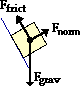There are only three forces present. The Fpar and Fperp are merely components of gravity; they are not separate forces. The normal force is perpendicular to the surface (drawn in blue).

 Useful Web Links Types of Forces || Drawing Free-Body Diagrams

[ #1 | #2 | #3 | #4 | #5 | #6 | #7 | #8 | #9 | #10 | #11 | #12 | #13 | #14 | #15 | #16 | #17 | #18 | #19 | #20 | #21 | #22 | #23 | #24 | #25 | #26 | #27 | #28 | #29 | #30 ]

### Part B: Conceptual Notions of Force and Motion

Express your understanding of concepts of force, acceleration, and motion by answering the following questions.

2. Which of the following are always true of an object that is at equilibrium? Include all that apply.

1. All the forces acting upon the object are equal.
2. The object is at rest.
3. The object is moving and moving with a constant velocity.
4. The object has an acceleration of zero.
5. There is no change in the object's velocity.
6. The sum of all the forces is 0 N.
7. All the forces acting upon an object are balanced.
 Answer: DEFG These (DEFG) statements are always true. Statements ABC might be true but are not always true.

 Useful Web Links Equilibrium and Statics || The Big Misconception

[ #1 | #2 | #3 | #4 | #5 | #6 | #7 | #8 | #9 | #10 | #11 | #12 | #13 | #14 | #15 | #16 | #17 | #18 | #19 | #20 | #21 | #22 | #23 | #24 | #25 | #26 | #27 | #28 | #29 | #30 ]

3. Which of the following are never true of an object that is at equilibrium? Include all that apply.

1. The object is accelerating.
2. The object is at rest.
3. The object is moving in a circle at constant speed.
4. All the forces acting upon the object are equal.
5. The object is in free-fall.
6. The object is falling and has reached its terminal velocity.
7. There is a net force acting upon the object.
8. The object is moving and moving with a constant velocity.
 Answer: ACEG An object that is at equilibrium can never be accelerating; its acceleration MUST be 0 m/s/s. Thus, A is an answer; and because an object in free-fall (E) and an object moving in a circle (C) are also accelerating, they must be counted as answers as well. (NOTE: an object moving in a circle is changing its direction and as such has an acceleration.) If there is a net force (G), then by definition the object is not at equilibrium. Choices B, D, F and H could be true of an object at equilibrium (though none of them are always true).

 Useful Web Links Equilibrium and Statics || The Big Misconception

[ #1 | #2 | #3 | #4 | #5 | #6 | #7 | #8 | #9 | #10 | #11 | #12 | #13 | #14 | #15 | #16 | #17 | #18 | #19 | #20 | #21 | #22 | #23 | #24 | #25 | #26 | #27 | #28 | #29 | #30 ]

4. Big Bubba has a mass of 100 kg on the earth. What is Big Bubba's mass on the moon where the force of gravity is approximately 1/6-th that of Earth's? ________ Explain or show your work.

 Answer: 100 kg Mass is the amount of matter present in the object and is independent of the weight of the object. The weight or force of gravity acting upon an object depends upon the mass and the acceleration of gravity (Fgrav = m•g). Because g is different on different planets and locations in the universe, the force of gravity or weight of an object will not always be the same. If Big Bubba goes to the moon, he will weigh less due to the decreased acceleration of gravity. However, he will still look the same, still have the same amount of matter, and still have a mass of 100 kg. KNOW the distinction between mass and weight.

 Useful Web Links Mass vs. Weight vs. Force of Gravity

[ #1 | #2 | #3 | #4 | #5 | #6 | #7 | #8 | #9 | #10 | #11 | #12 | #13 | #14 | #15 | #16 | #17 | #18 | #19 | #20 | #21 | #22 | #23 | #24 | #25 | #26 | #27 | #28 | #29 | #30 ]

5. Little Billie weight 360 N on Earth. What is Little Billie's mass on the moon where the force of gravity is approximately 1/6-th that of Earth's? ________ Explain or show your work.

 Answer: 37 kg (approx.) The acceleration of gravity on the moon is 1/6-th the value of earth's; this explains why the force of gravity is 1/6-th that of earth's. The force of gravity (Fgrav) on the moon is calculated using the equation Fgrav = m•g where g =1.6 m/s/s (on the moon). If Little Billie's weight on the moon is 1/6-th of 360 N, then Little Billie weighs 60 N on the moon. Inserting 60 N into the equation with 1.6 m/s/s as the value of g, Little Billie's mass can be calculated to be approximately 37 N. (Alternatively, simply find Billie's mass on earth from his weight of 360 N and then conclude that this mass value is not going to change when he goes to the moon.)

 Useful Web Links Mass vs. Weight vs. Force of Gravity

[ #1 | #2 | #3 | #4 | #5 | #6 | #7 | #8 | #9 | #10 | #11 | #12 | #13 | #14 | #15 | #16 | #17 | #18 | #19 | #20 | #21 | #22 | #23 | #24 | #25 | #26 | #27 | #28 | #29 | #30 ]

6. TRUE or FALSE:

An object which is moving rightward has a rightward force acting upon it.
 Answer: FALSE A rightward acceleration would require a rightward net force. But if an object is moving rightward and slowing down (a leftwards acceleration), then there is certainly not a rightward net force and possibly not even a rightward force at all. For instance, if a car is moving rightward and skidding to a stop (with wheels locked), then there is no rightward force upon the car. The only horizontal force is a leftward force of friction which serves to slow the car down. (If you're getting stuck on this question and ones like them, it might be time to read the page titled "The Big Misconceptsion"; use the link below.)

 Useful Web Links The Big Misconception

[ #1 | #2 | #3 | #4 | #5 | #6 | #7 | #8 | #9 | #10 | #11 | #12 | #13 | #14 | #15 | #16 | #17 | #18 | #19 | #20 | #21 | #22 | #23 | #24 | #25 | #26 | #27 | #28 | #29 | #30 ]

7. How much net force is required to keep a 5-kg object moving rightward with a constant velocity of 2 m/s? ________ Explain or show your work.

 Answer: 0 N If the velocity is constant, then the acceleration is 0 m/s/s and the net force is zero. A net force is only required in order to accelerate an object. Yes, this means that an object can be moving to the right and NOT have a rightward's net force. (If you're getting stuck on this question and ones like them, it might be time to read the page titled "The Big Misconceptsion"; use the link below.)

 Useful Web Links The Big Misconception

[ #1 | #2 | #3 | #4 | #5 | #6 | #7 | #8 | #9 | #10 | #11 | #12 | #13 | #14 | #15 | #16 | #17 | #18 | #19 | #20 | #21 | #22 | #23 | #24 | #25 | #26 | #27 | #28 | #29 | #30 ]

8. TRUE or FALSE:

For an object resting upon a non-accelerating surface, the normal force is equal to the force of gravity.
 Answer: FALSE In this instance, the normal force could be equal to the force of gravity. But all that we can conclusively know is that the all the vertical forces sum up to 0 N. If the object is upon an incline, then the normal force will not be equal to the force of gravity. Or if there is another force with an upward or downward component, then the normal force is not equal to the force of gravity.
[ #1 | #2 | #3 | #4 | #5 | #6 | #7 | #8 | #9 | #10 | #11 | #12 | #13 | #14 | #15 | #16 | #17 | #18 | #19 | #20 | #21 | #22 | #23 | #24 | #25 | #26 | #27 | #28 | #29 | #30 ]

9. An object rests upon an inclined plane. If the angle of incline is increased, then the normal force would _______.

 a. increase b. decrease c. remain the same
 Answer: B The normal force is equal to the perpendicular component of the weight vector. So Fnorm = Fperp = m•g•cos(angle). If the incline angle is increased, the cos(angle) decreases towards 0; thus, the normal force decreases as well. Of course, the extreme is when the angle is 90 degrees and there is no normal force.

[ #1 | #2 | #3 | #4 | #5 | #6 | #7 | #8 | #9 | #10 | #11 | #12 | #13 | #14 | #15 | #16 | #17 | #18 | #19 | #20 | #21 | #22 | #23 | #24 | #25 | #26 | #27 | #28 | #29 | #30 ]

10. Three pictures of equal weight (20 N) are hung by wires in three different orientations. In which orientation are the wires least likely to break? _______ Explain why.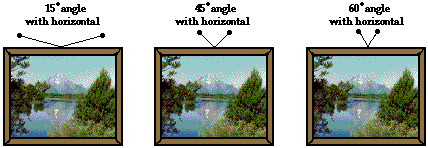Answer: 60-degree angle to the horizontal The tension in the wire will have the greatest impact upon its tendency to break. As the wire becomes most vertically oriented, the horizontal component of the tension force is reduced and the tension becomes less. The moral of the story -- to support the weight of a picture, one only needs to pull upwards, not leftwards and rightwards. Of course, the best arrangement would be two wires pulling completely vertically.

 Useful Web Links Equilibrium and Statics

[ #1 | #2 | #3 | #4 | #5 | #6 | #7 | #8 | #9 | #10 | #11 | #12 | #13 | #14 | #15 | #16 | #17 | #18 | #19 | #20 | #21 | #22 | #23 | #24 | #25 | #26 | #27 | #28 | #29 | #30 ]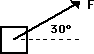11. A 50-N force is applied at an angle of 30 degrees north of east. This would be the same as applying two forces at

1. 43 N, east and 7 N, north
2. 35 N, east and 15 N, north
3. 25 N, east and 25 N, north
4. 43 N, east and 25 N, north
 Answer: D A 50-N force at 30 degrees would have two components - 43 N, east and 25 N, north. These two forces would be equal to the 50-N force at 30 degrees N of E. They are calculated as: Feast = (50 N)*cos(30 deg) Fnorth = (50 N)*sin(30 deg)

 Useful Web Links Resolution of Forces

[ #1 | #2 | #3 | #4 | #5 | #6 | #7 | #8 | #9 | #10 | #11 | #12 | #13 | #14 | #15 | #16 | #17 | #18 | #19 | #20 | #21 | #22 | #23 | #24 | #25 | #26 | #27 | #28 | #29 | #30 ]

12. Which one(s) of the following force diagrams depict an object moving to the right with constant speed? Write all possible answers (if any) in the blank: ________________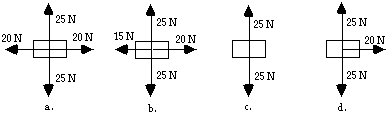Answer: AC Only A and C could have a constant speed. Both B and D would have an acceleration that is directed rightwards. Do not confuse acceleration, motion, and force. A rightwards acceleration requires that there be a rightwards net force, On the other hand, a rightwards motion could have no net force, a leftward net force or a rightwards net force; it depends upon whether the object is slowing down, speeding up or maintaining a constant speed. Only A and C show a balance of forces, so only A and C are moving with constant speed. If you're getting stuck on this question and ones like them, it might be time to read the page titled "The Big Misconception"; use the link below.

 Useful Web Links The Big Misconception

[ #1 | #2 | #3 | #4 | #5 | #6 | #7 | #8 | #9 | #10 | #11 | #12 | #13 | #14 | #15 | #16 | #17 | #18 | #19 | #20 | #21 | #22 | #23 | #24 | #25 | #26 | #27 | #28 | #29 | #30 ]

### Part C: Force-Mass-Acceleration Relationships

Use the approximation that g= ~10 m/s2 to fill in the blanks in the following diagrams.

 13.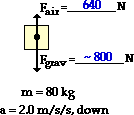Fgrav = m•g = ~800 N ∑Fy = may = (80 kg)•(2.0 m/s/s) ∑Fy = 160 N, down The Fgrav (down) and the Fair (up) must add up to 160 N, down. Thus, Fair must be smaller than Fgrav by 160 N. Fair = 640 N 14.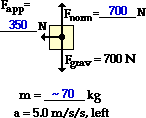Since  Fgrav = m•g, m can be calculated to be ~70 kg (m=Fgrav/g). Since ay = 0 m/s/s, Fnorm must equal Fgrav; so Fnorm = 700 N. ∑Fx = m•ax = (70 kg)•(5.0 m/s/s) ∑Fx = 350 N, left (Note that the ·Fx direction is always the same as the ax direction.) With Fapp being the only horizontal force, its value must be 350 N - the same as ·Fx. 15.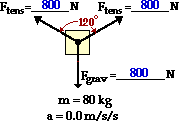Fgrav = m•g = ~800 N Since there are two forces pulling upwards and since the sign is hanging symmetrically, each force must supply an upwards pull equal to one-half the object's weight. So the vert pull (Fy) in each force is 400 N. The following triangle can be set up: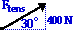Using trig, we can write: sin(30 deg.)=(400 N)/Ftens Solving for Ftens yields 800 N.

 16.A quick blank is Fgrav:  Fgrav = m•g = ~80 N Now resolve the 60-N force into components using trigonometry and the given angle measure: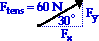Fx = 60 N•cos(30 deg) = 52 N Fy = 60 N•sin(30 deg) = 30 N Since the acceleration is horizontal, the sum of the vertical forces must equal 0 N. So Fgrav = Fy + Fnorm. Therefore Fnorm = Fgrav - Fy = 50 N. Knowing Fnorm and mu, the Ffrict can be determined: Ffrict = mu•Fnorm = 0.5*(50 N) = 25 N Now the horizontal forces can be summed: ∑Fx = Fx + Ffrict = 52 N, right + 25 N, left ∑Fx = 27 N, right Using Newton's second law, ∑Fx = m•ax So ax = (27 N)/(8 kg) = 3.4 m/s/s, right (3375... m/s/s) 17.The first step in an inclined plane problem is to resolve the weight vector into parallel and perpendicular components: Fpar = m•g•sin(angle) = (420 N)•sin(30 deg) = 210 N Fperp = m•g•cos(angle) = (420 N)•cos(30 deg) = 364 N The mass can be found as m = Fgrav/g m = Fgrav/g = (420 N)/(10 m/s/s) = ~42 kg The Fnorm acts opposite of and balances the Fperp. So Fnorm = Fperp = 364 N Knowing Fnorm and mu, the Ffrict can be determined: Ffrict = mu•Fnorm = 0.2*(364 N) = 73 N Now the forces parallel to the incline can be summed: ∑F|| = F|| + Ffrict = 210, down to left + 73 N, up to right ∑F|| = 137 N, down to left Using Newton's second law, ∑F|| = m•a|| So a|| = (137 N)/(42 kg) = 3.3 m/s/s 18.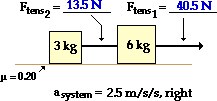Treating the two masses as a single system, it can be concluded that the net force on the 9-kg system is: ∑Fsystem = m•asystem = (9 kg)•(2.5 m/s/s) = 22.5 N, right The free-body diagram for the system is: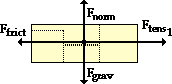The Fnorm supporting the 9-kg system is ~90 N. So the Ffrict acting upon the system is: Ffrict = mu• Fnorm = 0.20*(90 N) = 18 N, left So if ∑Fsystem = 22.5 N, right and Ffrict = 18 N, left, the rightward Ftens1 must equal 40.5 N. The Ftens2 force is found inside the system; as such it can not be determined through a system analysis. To determine the Ftens2, one of the individual masses must be isolated and a free-body analysis must be conducted for it. The 3-kg mass is selected and analyzed: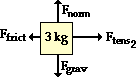The Fnorm and Fgrav balance each other; their value is ~30 N. The Ffrict on the 3-kg mass is: Ffrict = mu•Fnorm = 0.20*(30 N) = 6 N, left The net force on the 3-kg object is: ∑Fx = m•ax = (3 kg)•(2.5 m/s/s) = 7.5 N, right The horizontal forces must sum up to the net force on the 3-kg object; So ∑Fx = m•ax = Ftens2 (right) + Ffrict (left) 7.5 N, right = Ftens2 + 6 N, left The Ftens2 must be 13.5 N. 19.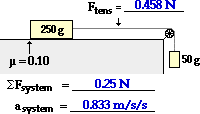Like most two-body problems involving pulleys, it is usually easiest to forgo the system analysis and conduct separate free-body analyses on the individual masses. Free-body diagrams, the chosen axes systems, and associated information is shown below.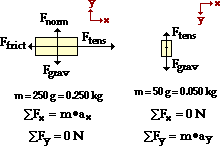Analyzing the Fx forces on the 250-g mass yields: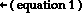max = Ftens - Ffrict Since Ffrict = mu•Fnorm and Fnorm = 2.5 N The Ffrict is (0.1)*(2.5 N) = 0.25 N. Substituting into equation 1 yields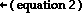(0.250 kg)•ax = Ftens - 0.25 N   Analyzing the Fy forces on the 50-g mass yields: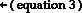may = Fgrav - Ftens Substituting m and Fgrav values into equation 3 yeilds: (0.050 kg)*ay = (0.500 N) - Ftens The above equation can be rearranged to: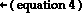Ftens = (0.500 N) - (0.050 kg)*ay Equation 4 provides an expression for Ftens; this can be substituted into equation 2: (0.250 kg)•ax = (0.500 N) - (0.050 kg)*ay - 0.25 N Now since both masses accelerate at the same rate, ax =ay and the above equation can be simplified into an equation with 1 unknown - the acceleration (a): (0.250 kg)•a = (0.500 N) - (0.050 kg)*a - 0.25 N After a few algebra steps, the acceleration can be found: (0.0300 kg)•a = 0.25 N a = 0.833 m/s/s Now that a has been found, its value can be substituted back into equation 4 in order to solve for Ftens: Ftens = (0.500 N) - (0.050 kg)*(0.833 m/s/s) Ftens = 0.458 N 20.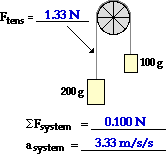This problem can most easily be solved using separate free-body analyses on the individual masses. Free-body diagrams, the chosen axes systems, and associated information is shown below.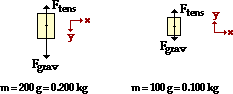Note that the positive y-axis is chosen as being downards on the 200-g mass since that is the direction of its acceleration. Similarly, it chosen as upwards on the 100-g mass since that is the direction of its acceleration. For the 200-gram mass, the sum of the vertical forces equals the mass times the acceleration:  Fgrav - Ftens = m•ay2.00 N - Ftens = (0.200 kg)•ay The same type of analysis can be conducted for the 100-gram mass: Ftens - Fgrav = m•ayFtens - 1.00 N = (0.100 kg)•ay Equation 2 can be rearranged to obtain an expression for the tension force:Ftens = (0.100 kg)•ay + 1.00 N This expression for Ftens can be substituted into equation 1 in order to obtain a single equation with acceleration (ay) as the unknown. The ay value can be solved for. 2.00 N -[(0.100 kg)•ay + 1.00 N] = (0.200 kg)•ay 2.00 N - 1.00 N = (0.200 kg)•ay + (0.100 kg)•ay 1.00 N = (0.300 kg)•ay ay = (1.00 N)/(0.300 kg) = 3.33 m/s/s Now with ay known, its value can be substituted into equation 3 in order to determine the tension force: Ftens = (0.100 kg)•ay + 1.00 N Ftens = (0.100 kg)•(3.33 m/s/s) + 1.00 N Ftens = 0.333 N + 1.00 N = 1.33 N 21.Like #20, this problem can most easily be solved using separate free-body analyses on the individual masses. Free-body diagrams, the chosen axes systems, and associated information is shown below. Note that in chosing the axis system, it has been assumed that object 1 will accelerate up the hill and object 2 will accelerate downwards. If this ends up to be false, then the acceleration values will turn out to be negative values.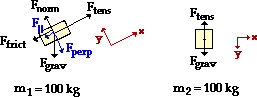Object 1 is on an inclined plane. The usual circumstances apply; their is no acceleration along what has been designated as the y-axis. Fnorm = Fperp = m•g•cos(theta) = 888.2 N The parallel component of Fgrav is F|| = m•g•sin(theta) = (100 kg)•(9.8 m/s2)•sin(25) F|| = 414.2 N The Ffrict value can be found from the Fnorm value: Ffrict = mu•Fnorm = (0.35)•(888.2 N) = 310.9 N The ∑Fx = m•ax equation can now be written: ∑Fx = m•ax Ftens - Ffrict - F|| = m•axFtens - 310.9 N - 414.2 N = m•ax (Note that the Ffrict and F|| forces are subtracted from Ftens since they are heading in the direction of the negative x-axis.) The above process can be repeated for object 2. The ∑Fy = m•ay equation can now be written: ∑Fy = m•ay Fgrav - Ftens = m•ay(980 N) - Ftens = m•ay The separate free-body analyses have provided two equations with two unknowns; the task at hand is to use these two equations to solve for Ftens and a. Equation 2 can be re-written as (980 N) - m•ay = Ftens Since both objects accelerate together at the same rate, the ax for object 1 is equal to the ay value for object 2. The subscripts x and y can be dropped and a can be inserted into each equation.(980 N) - m•a = Ftens Equation 3 provides an expression for Ftens in terms of a. This expression is inserted into equation 1 in order to solve for acceleration. The steps are shown below. (980 N) - m•a - 310.9 N - 414.2 N = m•a = 2•m•a 254.9 N = 2•(100 kg)•a 1.27 m/s2 = a The value of a can be re-inserted into equation 3 in order to solve for Ftens: Ftens = (980 N) - m•a =(980 N) - (100 kg)•(1.27 m/s2) Ftens = 853 N

 Useful Web Links Finding Acceleration || Net Force Problems Revisited || Inclined Planes

[ #1 | #2 | #3 | #4 | #5 | #6 | #7 | #8 | #9 | #10 | #11 | #12 | #13 | #14 | #15 | #16 | #17 | #18 | #19 | #20 | #21 | #22 | #23 | #24 | #25 | #26 | #27 | #28 | #29 | #30 ]

### Part D: Problem-Solving

22. A 945-kg car traveling rightward at 22.6 m/s slams on the brakes and skids to a stop (with locked wheels). If the coefficient of friction between tires and road is 0.972, determine the distance required to stop. PSYW

 Answer: 26.8 m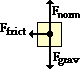Like most problems, this problem begins with a free-body diagram (as shown at right). Note that there is no rightwards applied force (a common mistake). Note also that the force of friction is the only force responsible for the acceleration (deceleration) of the car. The Ffrict value is the net force. So determining the acceleration involves finding Fgrav (Fgrav=m•g = 945•9.8 = 9261 N), Fnorm (the same as Fgrav= 9261 N), and Ffrict (Ffrict = mu•Fnorm = 0.972 • 9261 N = 9002 N). With the ∑Fx = 9002 N, the acceleration can be calculated (ax = ∑Fx/m = 9002 N/945 kg = 9.53 m/s/s.) This is a leftwards acceleration; ax will be assigned the numerical value of -9.53 m/s/s in the next part of this problem. Now in the kinematic part of this problem, the distance must be found using the known information (vf = 0 m/s, vo = 22.6 m/s and a = 9.53 m/s/s). Use the equation: vf2 = vo2 + 2 a d (0 m/s)2 = (22.6 m/s)2 + 2•(-9.53 m/s/s)•d d = [(22.6 m/s)2] / [2•(9.53 m/s/s)] = 26.8 m

 Useful Web Links Kinematic Equations and Problem-Solving || Finding Individual Forces

[ #1 | #2 | #3 | #4 | #5 | #6 | #7 | #8 | #9 | #10 | #11 | #12 | #13 | #14 | #15 | #16 | #17 | #18 | #19 | #20 | #21 | #22 | #23 | #24 | #25 | #26 | #27 | #28 | #29 | #30 ]

23. A student pulls a 2-kg backpack across the ice (assume frictionless) by pulling at a 30.0 degrees angle to the horizontal. The velocity-time graph for the motion is shown. Perform a careful analysis of the situation and determine the applied force. PSYW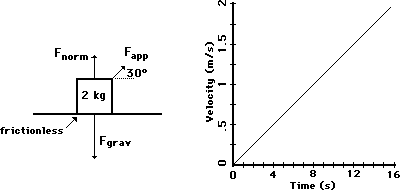Answer: 0.289 N The acceleration of the object can be found from the slope of the line on the v-t graph. The acceleration is: a = change in velocity/change in time = (2.0 m/s) / 16 s = 0.125 m/s/s. The net force is found from m•a; so Fnet = (2 kg)•(0.125 m/s/s) = 0.250 N.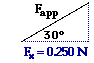The only force that can contribute to the net force is the applied force. The horizontal component of the applied force must therefore be equal to 0.250 N. A diagram depicting the relationship of the lengths of the side for this 30-60-90 triangle is shown at the right. The cosine function can be utilized to determine the magnitude of the tension force. cos(30 deg) = 0.250 N)/Fapp Solving for Fapp yields 0.289 N.

 Useful Web Links Net Force Problems Revisited || The Meaning of Shape for a v-t Graph

[ #1 | #2 | #3 | #4 | #5 | #6 | #7 | #8 | #9 | #10 | #11 | #12 | #13 | #14 | #15 | #16 | #17 | #18 | #19 | #20 | #21 | #22 | #23 | #24 | #25 | #26 | #27 | #28 | #29 | #30 ]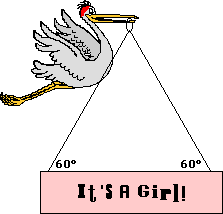24. After its most recent delivery, the infamous stork announces the good news. If the sign has a mass of 10.0 kg, then what is the tensional force in each cable?

 Answer: 56.6 N If the mass of the sign is 10.0 kg, then the weight of the sign is 98 N (Fgrav = m•g). This downward force of gravity must be balanced by the upward component of the tension. Thus, each cable must pull upwards with 49.0 N of force.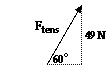In addition to the upward pull by the cable, there is also a horizontal pull. The tension force is the combined effect of these two componens. To determine the tension, set up a 60-30-90 triangle with a vertical component of 4.09 N as shown at the right. Then, use the sine function to solve for the hypotenuse of the triangle. The work is shown below: sin(60 deg.) = 49.0 N/Ftens Ftens = (49.0 N)/(sin 60 deg) = 56.6 N

 Useful Web Links Equilibrium and Statics

[ #1 | #2 | #3 | #4 | #5 | #6 | #7 | #8 | #9 | #10 | #11 | #12 | #13 | #14 | #15 | #16 | #17 | #18 | #19 | #20 | #21 | #22 | #23 | #24 | #25 | #26 | #27 | #28 | #29 | #30 ]

25. Splash Mountain at Disney World in Orlando, Florida is one of the steepest water plume rides in the United States. Occupants of the boat fall from a height of 100 feet (3.2 ft = 1 m) down a wet ramp which makes a 45 degree angle with the horizontal. Consider the mass of the boat and its occupants to be 1500 kg. The coefficient of friction between the boat and the ramp is 0.10. Determine the frictional force, the acceleration, the distance traveled along the incline, and the final velocity of the boat at the bottom of the incline. PSYW

 Answer: All numerical answers are rounded to two significant digits:  Ffrict = ~2100 N; a = ~5.5 m/s/s; d = ~44 m; vf = 22 m/s  This is a multi-part inclined plane problem. Like all problems, it should begin with a free-body diagram. If this is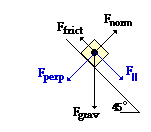where the difficulty lies for you, then take some time to review inclined planes. See help page. The friction force opposes the motion of the boat. If the + x direction is defined as down and along the incline (as shown at the right) then the net force can be computed by the expression F|| - Ffrict. The parallel component of the weight vector (m•g•sin theta) is 10395 N. The Ffrict force is computed by multiplying the coefficient of friction (0.10) by the normal force (Fnorm). The normal force is balancing Fperp (m•g•cos theta) and is equal to it. So Fnorm = 10395 N and Ffrict = 2079 N. The net force (Fnet = F|| - Ffrict ) is 8316 N; and the acceleration is Fnet/m or 5.54 m/s/s. Once the Newton's laws analysis has been completed and the acceleration determined, the kinematics portion of the problem can be tackled. First, determine the distance along the incline from the height of the hill and the angle of incline. The height of 100 ft is equivalent to 31.25 m; the distance along the incline is 44.19 m [found from (31.25 m)/sin (45)]. The final velocity is found using the acceleration, distance and a kinematic equation: vf2 = vo2 + 2•a•d vf2 = (0 m/s)2 + 2•(5.54 m/s/s)•(31.25 m) = 489.98 m2/s2 vf = 22 m/s

 Useful Web Links Kinematic Equations and Problem-Solving || Inclined Planes

[ #1 | #2 | #3 | #4 | #5 | #6 | #7 | #8 | #9 | #10 | #11 | #12 | #13 | #14 | #15 | #16 | #17 | #18 | #19 | #20 | #21 | #22 | #23 | #24 | #25 | #26 | #27 | #28 | #29 | #30 ]

26. At last year's Homecoming Pep Rally, Trudy U. Skool (attempting to generate a little excitement) slid down a 42.0 degree incline from the sports dome to the courtyard below. The coefficient of friction between Trudy's jeans and the incline was 0.650. Determine Trudy's acceleration along the incline. Begin with a free-body diagram. PSYW

 Answer: 1.82 m/s/s This is nearly an identical force analysis as the last problem except the mass is not known. When the mass is not known, the problem-solving strategy involves inserting m into the equation as an unknown variable and proceding with the solution. It is likely that in a subsequent step of the problem that the m will cancel and the accceleration can be determined without knowing m. The net force is found by the expression F|| - Ffrict. The parallel component of the weight vector is m•g•sin(theta). The Ffrict force is computed by multiplying the coefficient of friction (mu) by the normal force (Fnorm). The normal force is balancing Fperp and is equal to m•g•cos(theta). So Fnorm = m•g•cos(theta) and Ffrict = µ•m•g•cos(theta). The net force is m•g•sin(theta) - µ•m•g•cos(theta). The acceleration is Fnet/m or [m•g•sin(theta) - µ•m•g•cos(theta)]/m. Note that there is an m in both terms in the numerator and a m in the denominator. The masses cancel and the equation reduces to a = [g•sin(theta) - µ•g•cos(theta)] By substitution of the given values into the equation, it can be shown that a = 1.82 m/s/s.

[ #1 | #2 | #3 | #4 | #5 | #6 | #7 | #8 | #9 | #10 | #11 | #12 | #13 | #14 | #15 | #16 | #17 | #18 | #19 | #20 | #21 | #22 | #23 | #24 | #25 | #26 | #27 | #28 | #29 | #30 ]

27. Baldwin Young is conducting his famous toupee experiments. He tips his head at a given angle and determines the coefficient of static and kinetic friction between a toupee (which is probably his own) and his scalp. At an angle of just barely 17.5 degrees, the toupee begins to accelerate from rest. Then Baldwin lowers the angle to 13.8 degrees to observe that the toupee moves with a constant speed. Use this information to determine the coefficients of both static and kinetic friction. Begin with a free-body diagram. PSYW

 Answer: µstatic = 0.315; µkinetic = 0.246 A free-body diagram and a force analysis will yield the equation Ffrict = F|| for both the static and the kinetic situation. That is, when the object is at rest or moving down the incline at constant speed, the force down and parallel to the incline is balanced by the force which is up and parallel to the incline. The expressions for Ffrict and F|| can be substituted into this equation to yield µ•Fnorm = m•g•sin(theta) Knowing that Fnorm is equal in magnitude to Fperp, the expression for Fperp can be substituted into the equation for Fnorm. The new equation becomes µ•m•g•cos(theta) = m•g•sin(theta) The angles for both the static and the kinetic case are known and mu (coefficient of friction) is the unknown. So the equation can be re-arranged to µ = m•g•sin(theta)/m•g•cos(theta) The mass (m) and the acceleration of gravity (g) cancel from both the numerator and the denominator leaving sin(theta)/cos(theta) on the right side. This can be simplified again toµ = tan(theta) Equation 1 can be used to find the coefficient of friction for both the static and kinetic situation. The answers are as follows: µstatic = tan(17.5 deg.) = 0.315 µkinetic = tan(13.8 deg.) = 0.246

[ #1 | #2 | #3 | #4 | #5 | #6 | #7 | #8 | #9 | #10 | #11 | #12 | #13 | #14 | #15 | #16 | #17 | #18 | #19 | #20 | #21 | #22 | #23 | #24 | #25 | #26 | #27 | #28 | #29 | #30 ]

28. Consider the two-body system at the right. There is a 0.250-kg object accelerating across a rough surface. The sliding object is attached by a string to a 0.100 kg object which is suspended over a pulley. The coefficient of kinetic friction is 0.183. Calculate the acceleration of the block and the tension in the string. PSYW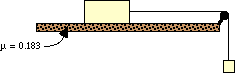Answer: a = 1.52 m/s/s ; Ftens = 0.83 N The solution to this problem begins by drawing a free-body diagram for each object. Note that the + x-axis for the 0.25-kg object is drawn in the direction that the object accelerates; and the + y-axis for the 0.10-kg object is drawn in the direction which it accelerates.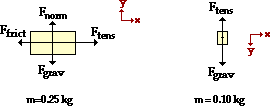Note that while friction acts upon the 0.25-kg object, it does not act upon the 0.10-kg object (since it is not being dragged across the surface). Newton's second law of motion (·F = m•a) can be applied to the motion of the 0.25-kg mass: ∑Fx = m•ax Ftens - Ffrict = m•ax The expressioin for Ffrict can be substituted into the equation.Ftens - µ•Fnorm = m•ax Since the vertical forces on the 0.250-kg object balance each other, it is known that Fgrav = Fnorm; so Fnorm = m•g = (0.250 kg)•(9.8 m/s/s) = 2.45 N. Since µ = 0.183, µ•Fnorm = 0.448 N. Equation 1 can now be re-written asFtens - 0.448 N = (0.250 kg)•ax Newton's second law of motion (∑F = m•a) can be applied to the motion of the 0.10o-kg mass: ∑Fy = m•ay Fgrav - Ftens = m•ay The expression for Fgrav (m•g) can be substituted into the equation. m•g - Ftens = m•ay (0.100 kg)•(9.8 m/s/s) - Ftens = m•ay 0.98 N - Ftens = m•ay The mass of the 0.100-kg object can be substituted into the equation to yield equation 3.0.98 N - Ftens = (0.100 kg)•ay Equations 2 and 3 each include an acceleration term - ax and ay. These acceleration values are the same since the system accelerates together; thus, ax = ay = a. An inspection of these two equations show that there are now two equations and two unknowns - Ftens and a. These unknown values can be solved for in the customary manner. First, equation 3 is rearranged to create an expression for Ftens:0.98 N - (0.100 kg)•a = Ftens This expression for Ftens is now substituted into equation 2.Ftens - 0.448 N = (0.250 kg)•a 0.980 N - (0.100 kg)•a - 0.448 N = (0.250 kg)•a This equation can now be solved for acceleration (a) as shown in the following steps. 0.980 N - 0.448 N = (0.250 kg)•a + (0.100 kg)•a 0.532 N = (0.305 kg)•a a = 1.52 m/s/s Now that a is known, its value can be substituted into equation 4 in order to solve for Ftens. 0.980 N - (0.100 kg)•a = Ftens 0.980 N - (0.10 kg)•(1.52 m/s/s) = Ftens 0.980 N - 0. 152 N = Ftens 0.830 N = Ftens
 Useful Web Links Double Trouble (a.k.a., Two-Body Problems)

[ #1 | #2 | #3 | #4 | #5 | #6 | #7 | #8 | #9 | #10 | #11 | #12 | #13 | #14 | #15 | #16 | #17 | #18 | #19 | #20 | #21 | #22 | #23 | #24 | #25 | #26 | #27 | #28 | #29 | #30 ]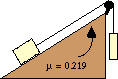29. Consider the two-body system at the right. A 22.7-N block is placed upon an inclined plane which is inclined at a 17.2 degree angle. The block is attached by a string to a 34.5-N block which is suspended over a pulley. The coefficient of friction is 0.219. Determine the acceleration of the block and the tension in the string. PSYW

 Answer: a = 3.95 m/s/s; Ftens = 20.6 N Like #21 and #28, this problem can most easily be solved using separate free-body analyses on the individual masses. Free-body diagrams, the chosen axes systems, and associated information is shown below. Note that in chosing the axis system, it has been assumed that object 1 will accelerate up the hill and object 2 will accelerate downwards on the back side of the hill. If this ends up to be false, then the acceleration values will turn out to be negative values.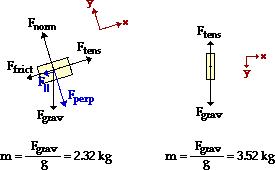The 22.7-N object is on an inclined plane. The usual circumstances apply; there is no acceleration along what has been designated as the y-axis. Fnorm = Fperp = m•g•cos(theta) = 21.7 N The parallel component of Fgrav is F|| = m•g•sin(theta) = (22.7 N)•sin(17.2) F|| = 6.71 N The Ffrict value can be found from the Fnorm value: Ffrict = µ•Fnorm = (0.219)•(21.7 N) = 4.75 N The ∑Fx = m•ax equation can now be written: ∑Fx = m•ax Ftens - Ffrict - F|| = m•axFtens - 4.75 N - 6.71 N = (2.32 kg)•ax (Note that the Ffrict and F|| forces are subtracted from Ftens since they are heading in the direction of the negative x-axis.) The above process can be repeated for the 34.5-N object. The ∑Fy = m•ay equation can now be written: ∑Fy = m•ay Fgrav - Ftens = m•ay(34.5 N) - Ftens = (3.52 kg)•ay The separate free-body analyses have provided two equations with two unknowns; the task at hand is to use these two equations to solve for Ftens and a. Equation 2 can be re-written as (34.5 N) - (3.52 kg)•ay = Ftens Since both objects accelerate together at the same rate, the ax for the 22.7-N object is equal to the ay value for the 34.5-N object. The subscripts x and y can be dropped and a can be inserted into each equation.(34.5 N) - (3.52 kg) •a = Ftens Equation 3 provides an expression for Ftens in terms of a. This expression is inserted into equation 1 in order to solve for acceleration. The steps are shown below. Ftens - 4.75 N - 6.71 N = (2.32 kg)•a (34.5 N) - (3.52 kg) •a - 4.75 N - 6.71 N = (2.32 kg)•a 23.0 N = (5.84 kg)•a 3.95 m/s2 = a The value of a can be re-inserted into equation 3 in order to solve for Ftens: Ftens = (34.5 N) - (3.52 kg) • (3.95 m/s2) Ftens = 20.6 N

 Useful Web Links Double Trouble (a.k.a., Two-Body Problems)

[ #1 | #2 | #3 | #4 | #5 | #6 | #7 | #8 | #9 | #10 | #11 | #12 | #13 | #14 | #15 | #16 | #17 | #18 | #19 | #20 | #21 | #22 | #23 | #24 | #25 | #26 | #27 | #28 | #29 | #30 ]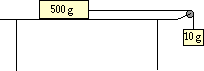30. A 10.0-gram mass is tied to a string.  The string is attached to a 500.0-gram mass and stretched over a pulley, leaving the 10.0-gram mass suspended above the floor.  Determine the time it will take the 10.0-gram mass to fall a distance of 1.50 meters if starting from rest. (No friction)

 Answer: t =  3.95 s  This problem involves a blending of Newton's laws and kinematics.  Newton's laws will have to be used to determine the acceleration;  then kinematics will have to be used to determine the time to fall 1.50 m from rest. The solution to this problem begins by drawing a free-body diagram for each object. Note that the + x-axis for the 500.0-gram object is drawn in the direction that the object accelerates; and the + y-axis for the 10.0-gram object is drawn in the direction which it accelerates.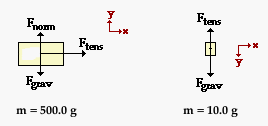Note that while friction acts upon the 500.0-gram object, it does not act upon the 10.0-gram object (since it is not being dragged across the surface). Newton's second law of motion (∑F = m•a) can be applied to the motion of the 0.5000-kg mass: ∑Fx = m•ax Ftens = m•ax Ftens  = (0.5000 kg)•ax Newton's second law of motion (∑F = m•a) can also be applied to the motion of the 0.0100-kg hanging mass: ∑Fy = m•ay Fgrav - Ftens = m•ay The expression for Fgrav (m•g) can be substituted into the equation. m•g - Ftens = m•ay (0.0100 kg)•(9.8 m/s/s) - Ftens = m•ay 0.098 N - Ftens = m•ay The mass of the 0.0100-kg object can be substituted into the equation to yield equation 2. 0.098 N - Ftens = (0.0100 kg)•ay Equations 1 and 2 include an acceleration term - ax and ay. These acceleration values are the same since the system accelerates together; thus, ax = ay = a. An inspection of these two equations show that there are now two equations and two unknowns - Ftens and a. These unknown values can be solved for in the customary manner. First, equation 2 is rearranged so as to express Ftens in terms of a.  This yields equation 3.Ftens = 0.098 N - (0.0100 kg)•ay Equations 1 and 3 both express Ftens in terms of a.  The right side of each equation is equal to Ftens ; so these two right sides can be set equal to each other to yield the following equation.    (0.5000 kg)•ax = 0.098 N - (0.0100 kg)•ay Since ax is equal to ay, the equation can be re-written as  (0.5000 kg)•a = 0.098 N - (0.0100 kg)•a where a is the horizontal and the vertical acceleration of the 500.0-gram and 10.0-gram masses. Now the above equation can be manipulated in order to solve for the value of a.    (0.5000 kg)•a + (0.0100 kg)•a = 0.098 N (0.510 kg)•a  = 0.098 N a = (0.098 N) / (0.510 kg) a = 0.1922 m/s/s Now that an acceleration has been determined, the time can be calculated using a kinematic equation.  The three known kinematic quantities are:   vi = 0 m/s a = 0.1922 m/s/s d = 1.50 m The following kinematic equation can be used to solve for time (t).  The substitutions and algebra are shown.   d = vi•t + 0.5 •a• t2 1.50 m = (0 m/s) • t  + 0.5•(.1922 m/s/s)•t2 1.50 m = (0.0961 m/s/s)•t2 t2 = (1.50 m) / (0.0961 m/s/s) t2 = 15.6087 s2 t = SQRT(15.6087 s2) t = 3.95 s

 Useful Web Links Double Trouble (a.k.a., Two-Body Problems) | Kinematic Equations and Problem-Solving

[ #1 | #2 | #3 | #4 | #5 | #6 | #7 | #8 | #9 | #10 | #11 | #12 | #13 | #14 | #15 | #16 | #17 | #18 | #19 | #20 | #21 | #22 | #23 | #24 | #25 | #26 | #27 | #28 | #29 | #30 ]

#### Navigate to:

Review Session Home - Topic Listing

Forces in 2 Dimensions - Home || Printable Version || Questions with Links

Answers to Questions:  All || #1-12 || #13-21 || #22-30

## You Might Also Like ...

Users of The Review Session are often looking for learning resources that provide them with practice and review opportunities that include built-in feedback and instruction. If that is what you're looking for, then you might also like the following:

The Calculator Pad includes physics word problems organized by topic. Each problem is accompanied by a pop-up answer and an audio file that explains the details of how to approach and solve the problem. It's a perfect resource for those wishing to improve their problem-solving skills.

Visit: The Calculator Pad Home | Calculator Pad - Forces in Two Dimensions

2. Minds On Physics the App Series

Minds On Physics the App ("MOP the App") is a series of interactive questioning modules for the student that is serious about improving their conceptual understanding of physics. Each module of the series covers a different topic and is further broken down into sub-topics. A "MOP experience" will provide a learner with challenging questions, feedback, and question-specific help in the context of a game-like environment. It is available for phones, tablets, Chromebooks, and Macintosh computers. It's a perfect resource for those wishing to refine their conceptual reasoning abilities. Part 2 of the series includes Forces in Two Dimensions.

Visit: MOP the App Home || MOP the App - Part 2